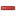# Sample Lessons

### We have released some sample lessons for you to try …

#### Check out our video guide on how to download a lessonYou must apply the theorem in order to find the missing value of an angle.

A.6.8.a Solve a < x ± e < b, a < dx < b, a < dx ± e < b, < > ≤ ≥ DYou have to solve the inequality in order to get a set of values for x.

N.18.1.b Percentage FractionYou have to convert each percentage to a fraction, leaving your answer in the simplest form.